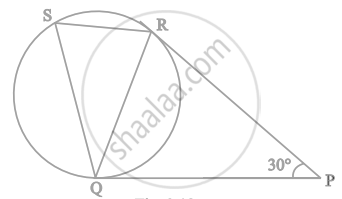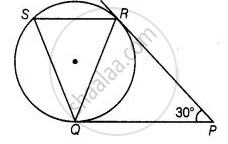# In figure, tangents PQ and PR are drawn to a circle such that ∠RPQ = 30°. A chord RS is drawn parallel to the tangent PQ. Find the ∠RQS. - Mathematics

Diagram
Sum

In figure, tangents PQ and PR are drawn to a circle such that ∠RPQ = 30°. A chord RS is drawn parallel to the tangent PQ. Find the ∠RQS.#### Solution

PQ and PR are two tangents drawn from an external point P.∴ PQ = PR  ....[The length of tangents drawn from an external point to a circle are equal]

⇒ ∠PQR = ∠QRP  ......[Angles opposite to equal sides are equal]

Now, In ΔPQR,

∠PQR = ∠QRP + ∠RPQ = 180°  .....[Sum of all interior angles of any triangle is 180°]

⇒ ∠PQR + ∠PQR = 30° = 180°

⇒ 2∠PQR = 180° – 30°

⇒ ∠PQR = (180^circ - 30^circ)/2 = 75^circ

Since, SR || QP

∴ ∠SRQ = ∠RQP = 75°   .....[Alternative interior angles]

Also, ∠PQR = ∠QSR = 75°  .....[By alternative segment theorem]

In ΔQRS, ∠Q + ∠R + ∠S = 180°  ......[Sum of all interior angles of any triangles is 180°]

⇒ ∠Q = 180° – (75° + 75°) = 30°

∴ ∠RQS = 30°

Concept: Number of Tangents from a Point on a Circle
Is there an error in this question or solution?

#### APPEARS IN

NCERT Mathematics Exemplar Class 10
Chapter 9 Circles
Exercise 9.4 | Q 7 | Page 110
Share